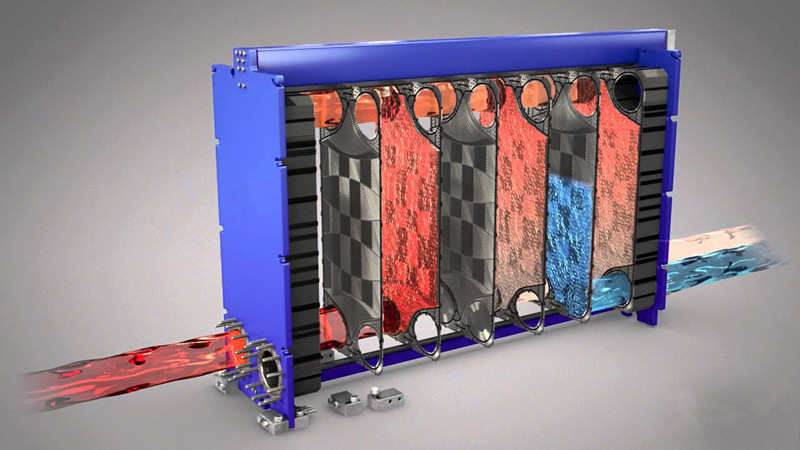# Plate Heat Exchanger DesignWorking Principle

Channels are formed between the plates and the corner ports are arranged so that two media flow through alternate channels. The heat is transferred through the plate between the channels, and complete counter-current flow is created for highest possible efficiency. The corrugation of the plates provides the passage between the plates, supports each plate against the adjacent one and enhances the turbulence, resulting in efficient heat transfer.

General Consideration

Plate Heat Exchanger Design Procedures

1 .Calculate duty, the rate of heat transfer required.

2. If the specification is incomplete, determine the unknown fluid temperature or fluid flow rate from a heat balance.

3. Calculate the log mean temperature difference, ΔTlm.

4. Determine the log mean temperature correction factor, Ft.

5. Calculate the corrected mean temperature difference ΔTm = Ft x ΔTlm.

6. Estimate the overall heat transfer coefficient.

7. Calculate the surface area required.

8. Determined the number of plates required = total surface area/area of one plate.

9. Decide the flow arrangement and number of passes.

10. Calculate the film heat transfer coefficients for each stream.

11. Calculate the overall coefficient, allowing for fouling factors.

12. Compared the calculated with the assumed overall coefficient. If satisfactory, say -10% to +10% error, proceed. If unsatisfactory, return to step 8 and increase or decrease the number of plates.

13. Check the pressure drop for each stream.